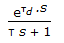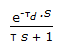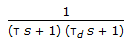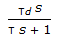# Chemical Engineering - Process Control and Instrumentation

### Exercise :: Process Control and Instrumentation - Section 3

11.

Starting temperature of optical radiation pyrometer is __________ °C.

 A. 800 B. 400 C. 1200 D. 1500

Explanation:

No answer description available for this question. Let us discuss.

12.

Liquid argon level in a pressurised storage tank (at 3 kg/cm2 ) is measured by a/an

 A. gage glass B. external float gage C. differential pressure gage D. none of these

Explanation:

No answer description available for this question. Let us discuss.

13.

Which of the systems having following transfer functions is stable?

 A. 1/(s2+ 2) B. 1/(s2-2s + 3) C. 1/(s2 + 2s + 2) D. exp(-20s)/(s2 + 2s-l)

Explanation:

No answer description available for this question. Let us discuss.

14.

What is the dynamic error in a critically damped second order instrument for a ramp input (At) ?

 A. 0.5 AT B. 2 AT C. AT D. 1.5 AT

Explanation:

No answer description available for this question. Let us discuss.

15.

The transfer function for a first order process with time delay is

 A.B.C.D.